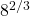# 3.1: Algebra Tips and Tricks Part V (Exponents)

$$\newcommand{\vecs}{\overset { \rightharpoonup} {\mathbf{#1}} }$$ $$\newcommand{\vecd}{\overset{-\!-\!\rightharpoonup}{\vphantom{a}\smash {#1}}}$$$$\newcommand{\id}{\mathrm{id}}$$ $$\newcommand{\Span}{\mathrm{span}}$$ $$\newcommand{\kernel}{\mathrm{null}\,}$$ $$\newcommand{\range}{\mathrm{range}\,}$$ $$\newcommand{\RealPart}{\mathrm{Re}}$$ $$\newcommand{\ImaginaryPart}{\mathrm{Im}}$$ $$\newcommand{\Argument}{\mathrm{Arg}}$$ $$\newcommand{\norm}{\| #1 \|}$$ $$\newcommand{\inner}{\langle #1, #2 \rangle}$$ $$\newcommand{\Span}{\mathrm{span}}$$ $$\newcommand{\id}{\mathrm{id}}$$ $$\newcommand{\Span}{\mathrm{span}}$$ $$\newcommand{\kernel}{\mathrm{null}\,}$$ $$\newcommand{\range}{\mathrm{range}\,}$$ $$\newcommand{\RealPart}{\mathrm{Re}}$$ $$\newcommand{\ImaginaryPart}{\mathrm{Im}}$$ $$\newcommand{\Argument}{\mathrm{Arg}}$$ $$\newcommand{\norm}{\| #1 \|}$$ $$\newcommand{\inner}{\langle #1, #2 \rangle}$$ $$\newcommand{\Span}{\mathrm{span}}$$$$\newcommand{\AA}{\unicode[.8,0]{x212B}}$$

## Exponents

When simplifying exponents, remember the exponentiation is just repeated multiplications. So if you have something like

$$x^3 x^7$$

This is three $$x$$s multiplied by seven $$x$$s, so that’s ten $$x$$s all multiplied together.

$$x^3 x^7 = x^{10}$$

Similarly, all these other rules don’t even have to be memorized if you just think about how repeated multiplication would work. But here they are anyway.

\begin{align*} A^x A^y & = A^{x + y} \\ \frac{A^x}{A^y} & = A^{x - y} \\ \left( A^x \right)^y & = A^{xy} \\ A^{-x} & = \frac{1}{A^x} \\ A^0 & = 1 \end{align*}

Some examples:

• $$\frac{e^{11}}{e^5 e^4}$$.

Here we have eleven $$e$$s, and we are taking away via division five of them then four of them. Hence we have two $$e$$s left over: $$\frac{e^{11}}{e^5 e^4} = \boxed{e^2}$$. Note that $$e$$ is a fundamental constant in mathematics, like $$\pi$$, equal to $$2.718281828459045\ldots$$ approximately, but we just use $$e$$ for the exact value.

• $$\frac{(A^4 B)^3}{(A B^4)^2}$$.

We see $$(A^4 B)^3 = A^{12} B^3$$. On bottom, we have $$(A B^4)^2 = A^2 B^8$$. This give $$\frac{A^{12} B^3}{A^2 B^8}$$. Once we get cancel two of the As and three of the Bs, we have $$\boxed{\frac{A^{10}}{B^5}}$$.

• $$\left(\frac{\sqrt{2} + \sqrt{3} + \sqrt{5} + \sqrt{7}}{\sqrt{104942}} \right)^{x - x}$$.

This looks ugly, but $$x - x = 0$$, and anything to the zeroth power is $$1$$. Hence the answer is $$\boxed{1}$$.

• $$a^{-5}a^2$$.

This combines as $$a^{-5 + 2} = a^{-3}$$, which we can also write as $$\boxed{\frac{1}{a^3}}$$.

## Fractional Exponents

One more rule before you go:. In other words, a fraction in the exponent is the same thing as taking a square root, cube root, 4th root, etc, depending on what the denominator is. Some examples:

•.

We see this is the same thing as $$\sqrt{25}$$, which is $$\boxed{5}$$.

•.

This is the same thing as $$\left( \sqrt{8}\right)^2$$. We see that $$\sqrt{8} = 2$$, and hence we do $$2^2 = 4$$. So.

This page titled 3.1: Algebra Tips and Tricks Part V (Exponents) is shared under a CC BY 4.0 license and was authored, remixed, and/or curated by Tyler Seacrest via source content that was edited to the style and standards of the LibreTexts platform; a detailed edit history is available upon request.